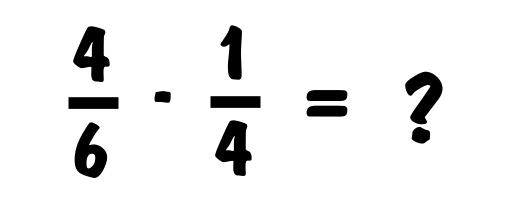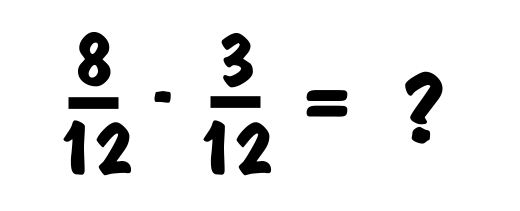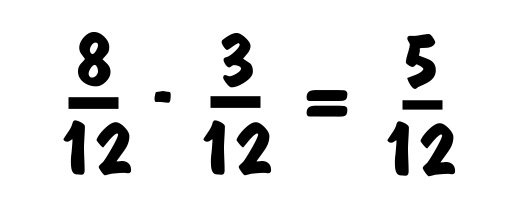How To Subtract FractionsSubtracting fractions is pretty easy. We will teach you how to subtract fractions with this example:To subtract fractions, you must first make the the denominators the same. The denominator is the bottom number. To make the denominator the same, you look for the lowest common denominator. The lowest common denominator is the smallest whole number that is divisible by each of the denominators. In this case it is 12. To make both denominators 12, find out what you have to multiply each denominator by to get 12, then use that number to muliply the numerators (top numbers) to keep the fraction intact.After you multiply the above to get the common denominator, then you get the following:Then you simply leave the denominator, and subtract the second numerator from the first numerator and you have your answer:More information from Research Maniacs: Fraction Calculator The best online fraction calculator. How To Add Fractions Learn How To Add Fractions here! How To Multiply Fractions Learn How To Multiply Fractions here! How To Divide Fractions Learn How To Divide Fractions here!

Copyright  |   Privacy Policy  |   Social Media  |   Disclaimer  |   Advertise